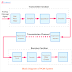Pulse Code Modulation(PCM) is a digital modulation technique where the analog signal is coded into digital pulses. Here, we will see the block diagram of the PCM system or Pulse Code Modulation system to understand its working principle. For a long transmission system, a carrier signal is used and according to the actual data-carrying signal, the carrier signal is modulated. So, in simple words, the process of varying parameters of a carrier signal is known as modulation. There are various types of modulation techniques are available related to analog and digital systems. The PCM or Pulse Code Modulation is one of them.

## PCM Block Diagram

First, of all let's see the block diagram of the Pulse Code Modulation system.

## PCM Working Principle

If we know the function of each part of the system then we can easily understand the working principle of PCM. Now let's see the important parts or blocks of the PCM system are,

### 1. Low Pass Filter

As its name suggests, the low pass filter will pass only low-frequency signals. So, the analog signal is applied to the input of the low pass filter and the low pass filter will block the high-frequency signal and pass the low-frequency signal to the next stage. A frequency is set as per the frequency of the message signal in the filter circuit with respect to which the signal will be cut off.

### 2. Sampler

The sampler circuit helps to take sample data from the message signal at the instantaneous value. It can be called a process of cutting the continuous signal into discrete from. The low-frequency analog signal comes from the filter circuit to the sampler circuit and it is sampled at regular intervals. Generally, the sampling rate or sample frequency is twice than the highest frequency component of the message signal. So, fs ≥ 2fm
Here, Fs= sampled frequency, and Fm = message signal frequency

### 3. Quantizer

The quantizer is a circuit that does quantizing or compress or reduces the no of samples. It removes the excessive bits from the sampled signal provided by the sampler circuit. Basically, it confines the data and makes rounds off each sample. Actually, it gives the shape of digital bis or digital pulse from the sinusoidal shape. For example, if the voltage is 5V then it is considered as high but if the voltage is 0V, then it is considered as low. So the quantizer can make the 4.8V to 5V or 0.5V to 0V or remove the 3V signals.

There are two types of quantizing processes - uniform quantizing and non-uniform quantizing. In the uniform quantizing process there is a uniform spacing between the levels whereas, in the non-uniform quantizing, the spacing between the levels is non-uniformed.

### 4. Encoder

The encoder circuit converts the quantizing signal into binary codes. So this unit generates the actual digital encoded signals. This digital signal is consists of binary pulses and they act as modulated output from the transmitter section of the whole system.

### 5. Regenerative Repeater

The regenerative repeater is placed in both ends of the transmission channel sending and receiving end. at the sending end, it makes ready the signal for long-distance transmission. And at the receiving end, it removes noise from the signal and gives reshape to the signal to get the actual signal. There may be multiple numbers of regenerative repeaters for long-distance transmission.

### 6. Transmission Channel

A transmission channel is a medium or path for data transmission. Through this channel or medium data or signal is transmitted.

### 7. Regenerator Circuit

At the receiving end of the transmission channel, the regenerator circuit is used. It also works the same as the regenerator repeater. It removes the noise and distortion from the signal received from the transmission channel and generates the actual signal sent.

### 8. Decoder

The decoder circuit converts the received digitally coded binary signal into the original signal. This circuit also demodulates the signal. The generative circuit and decoder both are responsible to convert the received digital signal into an analog signal.

### 9. Reconstruction Filter

The filter at the receiving end of the system is known as the reconstruction filter. The reconstruction filter provides the actual original signal sent by the sender.

Pulse Code Modulation(PCM) Block Diagram, Working PrincipleReviewed by Author on February 02, 2022 Rating: 5